## Quantization of the Oscillators

To summarize the result of the calculations of the last section we have the Hamiltonian for the radiation field.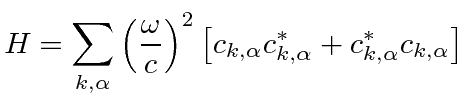Soon after the development of non-relativistic quantum mechanics, Dirac proposed that the canonical variables of the radiation oscillators be treated likeandin the quantum mechanics we know. The place to start is with the commutators. The coordinate and its corresponding momentum do not commute.For example. Coordinates and momenta that do not correspond, do commute. For example. Different coordinates commute with each other as do different momenta. We will impose the same rules here.By now we know that if theand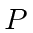do not commute, neither do theandso we should continue to avoid commuting them.

Since we are dealing with harmonic oscillators, we want to find the analog of the raising and lowering operators. We developed the raising and lowering operators by trying to write the Hamiltonian as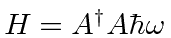. Following the same idea, we get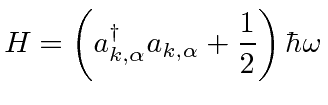This is just the same as the Hamiltonian that we had for the one dimensional harmonic oscillator. We therefore have the raising and lowering operators, as long as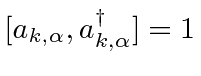, as we had for the 1D harmonic oscillator.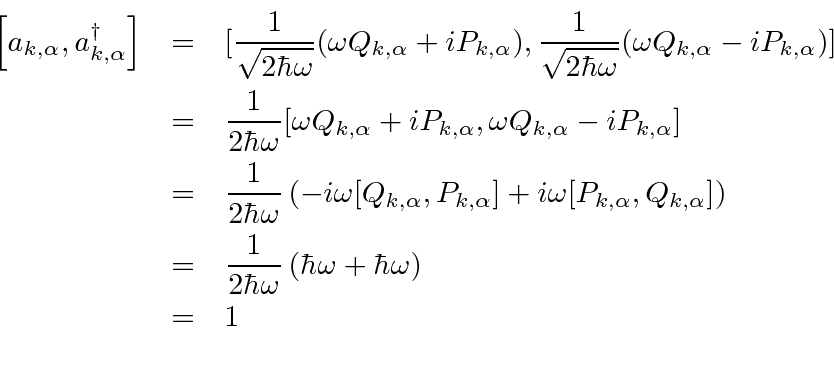So these are definitely the raising and lowering operators. Of course the commutator would be zero if the operators were not for the same oscillator.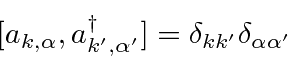(Note that all of our commutators are assumed to be taken at equal time.) The Hamiltonian is written in termsandin the same way as for the 1D harmonic oscillator. Therefore, everything we know about the raising and lowering operators applies here, including the commutator with the Hamiltonian, the raising and lowering of energy eigenstates, and even the constants.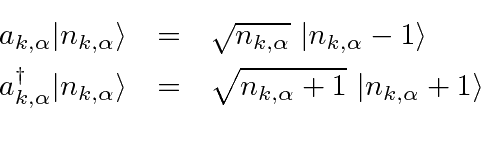Thecan only take on integer values as with the harmonic oscillator we know.

As with the 1D harmonic oscillator, we also can define the number operator.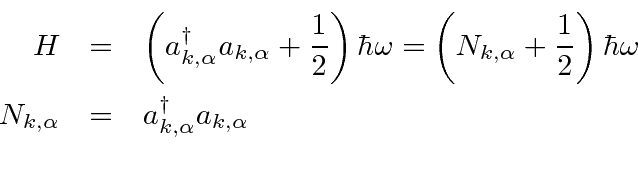The last step is to compute the raising and lowering operators in terms of the original coefficients.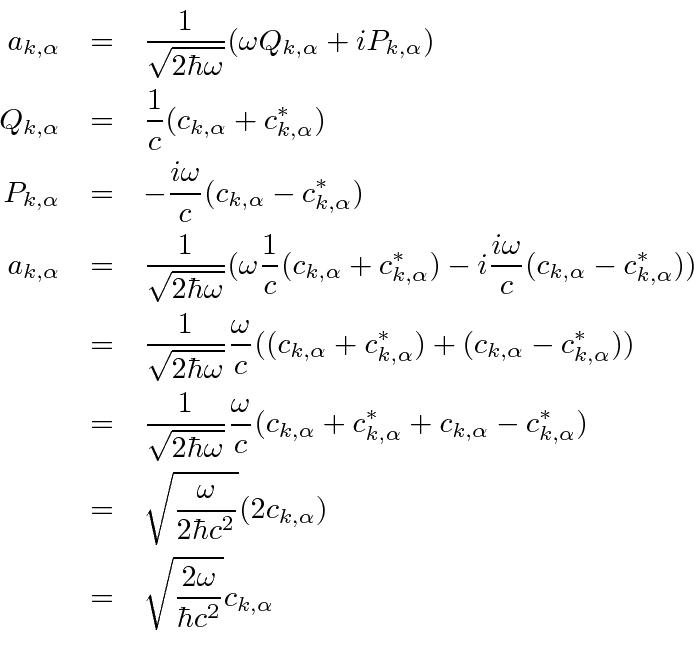Similarly we can compute thatSince we now have the coefficients in our decomposition of the field equal to a constant times the raising or lowering operator, it is clear that these coefficients have themselves become operators.

Jim Branson 2013-04-22